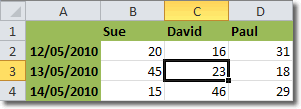Planet For Application Life Development Presents MY IT WorldExplore and uptodate your technology skills...

Excel - Cell References

A cell reference refers to a particular cell or range of cells in your worksheet. Cell references are useful for Excel to identify data for use in formulas to calculate results based on your data.

You can easily tell what the cell reference is by looking at the cross section of the row and column for that cell. For example, the cell below occurs at the cross section of column C and Row 3.The cell reference is therefore C3 and we can use C3 to refer to the data in that cell.

We can use cell references to refer to:

• Data from one cell in your worksheet.
• Data that is contained in different areas of your worksheet.
• Data in cells on other worksheets in the same workbook

Cell References For A Range Of Cells

C3 refers to the contents of just one cell. What if we want to refer to a range of cells?

Consider the following range of cells.So far we have looked at cell references on the sme worksheet. Let’s now look at references to cells on different worksheets.

Cell References On Other Worksheets

Suppose that we are using two worksheets in the same workbook: Sheet1 and Sheet2. We are creating a formula in Sheet1 that uses data in cell E12 on Sheet2. The reference to this cell is simply Sheet2!E12. Don’t forget the exclamation mark after the worksheet name.

For example, let’s suppose that we are working on Sheet1 and we want to sum the values of cells A1 to A10 on Sheet2. The formula would be

=SUM(Sheet2!A1:A10)

Dont worry too much about how to create formulas, the important part is the Sheet2!A1:A10. Note that the Sheet! bit tells Excel that the cells are on Sheet2 and the A1:A10 bit tells Excel that it’s dealing with the range of cells A1 to A10.